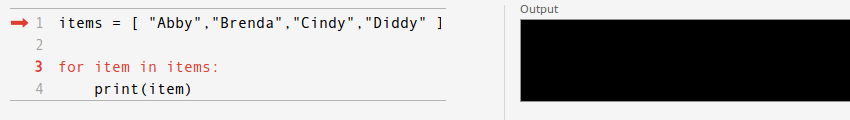# Loops: For loop, while loop

Code can be repeated using a loop. Lines of code can be repeated N times, where N is manually configurable. In practice, it means code will be repeated until a condition is met. This condition is usually (x >=N) but it’s not the only possible condition.

Python has 3 types of loops: for loops, while loops and nested loops.
Related Course:
Complete Python Bootcamp: Go from zero to hero in Python 3## For loop

We can iterate a list using a for loop

Visualization of for loop:The for loop can be used to repeat N times too:

## While loop

If you are unsure how many times a code should be repeated, use a while loop.
For example,

## Nested loops

We can combine for loops using nesting. If we want to iterate over an (x,y) field we could use:

Nesting is very useful, but it increases complexity the deeper you nest.

## 21 thoughts on “Loops: For loop, while loop”

1.- April 22, 2017

Hi Frank.
There is a little mistake in the “While loop” example. It should be:

` guess = int(input("Guess the number: "))`

Otherwise, the compiler will compare between string and integer, and ‘5’!=5.
Except it – excellent!

1.- April 22, 2017

Thanks Sagi!
I’ve updated the article.

2.- January 28, 2016

whats wrong with this code not generating primes as i expected

`i=3num=int(input("enter the no. upto which u want the primes: "))arr=print(2)while i&lt;num: j=0 while j&lt;len(arr): if i%arr[j]==0: i=i+1 j=len(arr) else: j=j+1 print i arr[len(arr)]=i`

1.- January 30, 2016

The conditions in the while loops are never true. I fixed your code:

`num=int(input("enter the no. upto which u want the primes: ")) arr=print(arr) i = 2while max(arr) < num:  prime = True for p in arr: if i % p == 0: prime = False break  if prime: arr.append(i)  i = i + 1   print(arr) print(arr)`

You can see the prime numbers being added to the array in the loop.

3.- December 3, 2015

why are you using str() in print statement i am getting even i used variable directly

1.- December 3, 2015

sometimes that may lead to problems as they have different datatypes,just being cautious.